## Introduction

The characterization of the architecture of economic and financial networks is gaining increasing importance1,2. Indeed, the recent economic turmoil has raised a broad awareness that the financial system should be regarded as a complex network whose nodes are financial institutions and links are financial dependencies3. In this perspective, systemic risk is meant here as the risk of a systemic default, i.e. the default of a large portion of the financial system. It can be quantified and measured from the analysis of the dynamical evolution of the nodes and from the structure of the network4,5,6,7. The main open question regarding financial networks concerns the determination of the so-called “systemically important” financial institutions, namely, the ones that, if defaulting, can trigger a systemic default and are thus to be considered “too-big-to-fail”. From a network science perspective, this question is related to the concept of recursive centrality measures such as eigenvector centrality and PageRank. It is also related to the more general issue of the controllability of a complex network8. However, the investigation of how financial networks function and how systemic risk emerges is only at the beginning. The scarcity of data, due to confidentiality constraints, has limited so far the study to few national datasets9,10,11,12. The goal of this paper is to show how network science can contribute to a quantitative assessment of systemic risk. To this end, we analyse a unique and very relevant dataset by means of a novel indicator of systemic importance.

In the US, the financial crisis reached a peak in the period March 2008 - March 2010. During this time, many US and international financial institutions received aid from the US Federal Reserve Bank (FED) through emergency loans programs, including the so-called “FED Discount Window”. The amount and the recipients of these loans were not disclosed until very recently (see more details in Supplementary Information, SI, Section 1-2). This data represents, to our knowledge, the first data set, publicly available, on the daily financial exposures between a central bank and a large set of institutions over several months. The data was previously analysed mainly from the point of view of accounting practice and conflicts of interests13. Here, we instead present an analysis from the perspective of complex financial networks and systemic risk.

The contributions of this paper are the following. We first analyse the portfolio of loans granted by the FED over time, both in terms of concentration and fragility. We then investigate the distribution of outstanding debt across institutions and across time. We also combine the FED dataset with data on equity investment relations among these institutions and we analyse the structure of the network of dependencies among the institutions that received funding. Finally, in order to estimate the systemic importance of the various institutions, we introduce Debt Rank. This is a novel measure, akin to feedback centrality, that takes into account in a recursive way the impact of the distress of one or more institutions to their counterparties across the whole network.

## Results

### Credit concentration and fragility

We start our analysis of the dataset (see Methods) from the sum of the outstanding debt across institutions, which represents the total exposure of the FED, i.e. its total potential loss in case of default of the borrowers. This number rose very sharply in November of 2008, up to around USD 1.2 trillion, from where, in the following 12 months, it steadily decreased down to few billions (Fig. 1a). As a measure of concentration of the credit portfolio, we compute the Herfindhal index, H, of the outstanding debts (see Methods). As shown in Fig. 1, H remains around 0.05, meaning that, despite the large variation in the size of the exposure, the number of leading borrowers remained approximately around 20. What did not remain constant over time is the fragility of the leading borrowers, defined as debt-to-equity ratio. The weighted average, Φ(t), of the fragility, which reflects the fragility of the largest borrowers, displays peaks in correspondance to the major events, such as the Bear Sterns case (March of 2008) and the drop in stock market value as of March of 2009 (Fig. 1b). Remarkably, when the total exposure of the FED still amounted to 900 billions, the debt of the leading borrowers was more than 6 times larger than their market capitalization.

### Debt distribution

The dataset provides a unique opportunity to study the distribution of the outstanding debt across institutions and across time. Based on the behaviour of the total FED exposure, we divide the time span of the data into 3 phases (see caption Fig. 2a). In principle, we could study the daily evolution of the distribution. In practice, on a single day the number of institutions with debt to the FED is not always large enough to reveal its underlying distribution. To improve the statistics, we pool the values of debt over intervals of 30 days, after checking for the homogeneity of the debt time series within those intervals (see also Sec. 2 of SI). As shown in the inset of Fig. 2a, we choose ten representative such intervals so that each phase is covered. Fig. 2a shows the complementary cumulative distribution of outstanding debt, computed in each of the 30-day intervals. The values of debt are rescaled by the total debt across institutions. The hypothesis that the distribution of debt across institutions is a power law is rejected by statistical tests in all periods (see also SI, Sec. 2.1). This contrasts with what was found in previous studies on the distribution of debt9,14 and more in general in various economic contexts15,16. However, here we are in presence of emergency loans for which not every firm is eligible. It is thus likely that small institutions are under-represented in this dataset and that there is also an upper limit on the loans, which would explain the observed deviation from a power law (see also SI, Sec. 2).

Statistical tests suggest that the functional form of the distributions is the same across periods and that their parameters are more similar within the same market phase (see also SI, Sec. 2). This can be seen also in Fig 2.a where the curves in blue and violet cluster together. This is a remarkable finding, in particular during the third phase, since the total amount of debt to the FED varied in time by almost one order of magnitude (from USD 1200 billion in January of 2009 to less than USD 200 billions in October of 2009). A trivial explanation of the scaling behaviour would be that at each point in time the FED lends to all institutions in the same proportion and that only the total amount varies. The analysis of the outstanding debt pattern over time across the top 100 borrowers, shown in Fig. 2b, rules out this explanation. We find that although about 30 institutions reached their respective peak of debt in the same period, many others had their peak few months earlier or later. Moreover, while for some institutions the debt declined rapidly, for others it declined slowly. Given the heterogeneity across the debt patterns (Fig. 2b), the similarity of the debt distributions seems to be an emerging property of the system. Further evidence of this property comes from complementary data on the FED balance sheet. We find that the equivalent amount that was paid back by the institutions reappears on the FED balance sheet as mortgage backed securities (see SI, Fig. 1b). This suggests that as banks started to pay back their debt, the FED began to buy from them the toxic assets which were at origin of the crisis (more details in SI, Section 3).

### Network analysis

In a network perspective, nodes represent institutions, while directed edges represent lending relations weighted by the amount of the outstanding debt. The network has a simple structure (a star with the FED in the center, see also SI, Fig. 2a-11a), although it is known that these institutions had also many financial dependencies among each other. Indeed, it is part of the normal business of financial firms to invest in other firms by utilizing various lending mechanisms9,10,11,12 and by acquiring equity stakes (although these forms of investment cannot be regarded as equivalent). Unfortunately, information on the former type of investment is not available because of confidentiality issues, especially because these are global firms from different countries. However, previous work has shown that the equity investment network of transnational corporations (TNC) has a bow-tie structure with financial firms in the core forming a tightly connected structure3.

We thus match the FED dataset with data on equity investment relations at the end of 2007 (i.e. at the beginning of the FED discount window data time span). We then focus on the 22 institutions that received, on average over the 10 periods, more than USD 5 billion. The top borrowing institutions accounted, at the peak of the crisis, for a total of USD 804 billion out of USD 1.2 trillion of funding. Interestingly, all these institutions turn out to be in the core (i.e., the largest strongly connected component) of the TNC network mentioned above. Moreover, the subnetwork of these 22 institutions is itself strongly connected (which would not need to be true). This means that each of the 22 institutions in the network can reach any other via a directed path. The overwhelming majority of pairs of nodes (99.1%) are at distance 1 or 2 from each other. The structure is relatively homogeneous with a high density of connections. The average out-degree and in-degree are, respectively, 12.1 ± 5.7 and 12.1 ± 3.2 (the average must coincide although the network is not symmetric). The density of links is 0.58. The k-core analysis yields a core number equal to 8 for all nodes except 3, with an average of 7.7. This finding suggests, in the lack of alternative information, to take as a proxy of the financial dependencies among institutions the network of their mutual equity investments, appropriately normalised (see Methods).

### DebtRank and systemically important institutions

In order to estimate the impact of a node on the others, we then introduce DebtRank, a novel measure inspired by feedback centrality, that takes recursively into account the impact of the distress of an initial node across the whole network. DebtRank of node i, denoted as Ri, is a number measuring the fraction of the total economic value in the network that is potentially affected by the distress or the default of node i (see Methods). DebtRank can be used to construct a ranking, but it is not itself the rank of the node. Its computation differs from the methods based on the default cascade dynamics11,12,17 in which, below the threshold no impact is propagated to the neighbors18 (see SI, Section 3.1). Feedback centrality measures19 have found successful applications in many domains ranging from rankings in the world-wide-web (e.g. PageRank) to corporate control in economic networks3. Feedback centrality has a physical analogy with the in-flow in a non-homogeneous diffusion process (see SI, Section 3.1). In presence of a cycle in the network there is an infinite number of reverberations of the impact of a node to the others and back to itself, which leads to no simple and measurable economic interpretation. DebtRank overcomes this problem by only allowing for walks that do not visit the same edge twice.

We apply the computation of DebtRank on the network of the 22 top borrowers of the FED by carrying out two experiments. In the first one, for each node i, we compute DebtRank in the case of its default (i.e., ψi = 1, see Methods). Notice that in the impact matrix used by DebtRank we take into account the level of market capitalization of the nodes (see Methods), thus obtaining a set of values of Ri in each period. The diagrams in Fig. 3 display the structure of the network and allow, at the same time, to compare the values of DebtRank of any pair of institutions. A high value of DebtRank corresponds to a more central location of the node. Therefore, the terms “DebtRank” and “centrality” will be used interchangeably in the following. As it can be seen from the figure, at the peak of the crisis all nodes became more central, meaning that the default of each of them would cause a larger economic loss in the network. Figure 4 shows instead how the DebtRank of each institution varies with its total asset, relative to the sum of total assets in the network. The color of the bubbles reflects the fragility of the institutions (i.e. debt over equity ratio). We observe that towards the peak of the crisis most institutions have high level of fragility and in addition high values of DebtRank. This implies that each bank was more likely to default on its own, and, moreover, that in case of default, it would cause a larger loss. We find that even in a conservative scenario (e.g. we assume that in the first months of 2008 each bank had enough core capital to withstand at least the default of 5 counterparties, α = 0.2, see also Methods), most of the 22 institutions become very systemically important at the peak of the crisis (Fig. 3b). In period 1, all institutions have a DebtRank below 0.25 with an average value of 0.08 ± 0.06 (Fig. 4a) and are not very central (Fig. 3a). In contrast, in period 4 (average 0.52 ± 0.20), many institutions are able, alone, to affect more than the 70% of the total value in the network (Fig. 4b), despite the fact that in terms of asset size they represent less than 10% of the total.

In the second experiment, we compute DebtRank in the situation in which none of the nodes in the network defaults initially but all are at level ψ < 1 of distress (see Methods). We refer to this case as Group DebtRank. We find that the initial shocks always generate an impact larger than the shocks themselves even in the periods preceding the peak of the crisis. For instance, with ψ = 0.1, i.e. an initial shock of 10%, the total loss varies across periods from a minimum of 22% to a maximum of 65% (see SI, Section 3.6). Notice, that the mechanism differs from the contagion found in epidemic spreading20 and other contact processes. Here, the impact of an institution i to another, j, depends both on the relative exposure of j to i and on the ratio between such exposure and the capital buffer of i (see Methods). It follows that the impact of i over j can be larger then the relative exposure of j to i (the impact matrix is not column stochastic). Indeed, financial institutions, by seeking for profit, tend to be highly leveraged, i.e., to have small capital compared to total assets. This is how the system can end up in a situation in which a small loss can cause a systemic default.

It is important to emphasise that there is more to DebtRank than size. The correlation between asset size and DebtRank is always lower than 0.4 and decreases towards the peak of the crisis (see SI, Section 3.7). We have also compared DebtRank with the measures of systemic imault cascade dynamics and with two feedback centralities, including the classic eigenvector centrality. In a nutshell, all measures differ in the timing and in the magnitude of their response. Although the ranking provided by the four measures are similar (implying that these measures are consistent among each other), DebtRank is the only one that delivers a clear response well before the peak of the crisis (see SI, Sec. 3.5). This feature makes it a better candidate as a possible early-warning indicator. Moreover, DebtRank has the precise meaning of economic loss, measured in dollars, caused by the distress or default of a node.

## Discussion

In this paper, we presented the first complex networks analysis of the controversial FED emergency program dataset. We find that, while the number of leading borrowers to the FED only varied between 10 and 30, the fragility of the portfolio reflected closely the major events of distress in the market. We also find that the distribution of debt across institutions has the same functional form over time. In order to estimate the systemic importance of a node, or a group of nodes, we introduce DebtRank, a novel metric that, similar to PageRank and other feedback centrality measures, takes into account recursively all the paths in the network. However, differently from these, DebtRank avoids the infinite reverberation by excluding the walks in which edges are repeated. By matching the FED data with data on equity investments relations in the same period, we find that the largest borrowers were interconnected in a dense network. Each of them was very central and could impact significantly every other node in only one or two steps. Moreover, we find that a small shock to the system as whole could get amplified by the many paths in the network into a systemic default. These findings should be regarded with caution since the network of impact used for the experiments is a proxy of the real, unknown, one. However they demonstrate what kind of insights can be gained with our methodology. The work contributes to the understanding of financial networks, but the methodology is relevant to the field of Complex Networks in general, since it can be applied to detect systemically important nodes in any directed and weighted network.

The methodology is also relevant to a broader audience of regulators and risk management practitioners since it provides a quantitative assessment of the systemically important financial institutions. In this respect, our results suggest that the current public discussion on too-big-to-fail institutions should be broadened to the network-theory notion of too-central-to-fail, so to account for the existence of densely connected cores in financial networks.

## Methods

### Data

Data consists of daily time series of outstanding debt and market capitalization of 407 institutions in a period of 1004 days spanning from August 2007 to June 2010. Outstanding debt refers here to the amount owed by an institution to the FED as a result of the emergency loans granted by the FED via the so-called Discount Window and various emergency programs. This means that these were loans for which the FED was lender-of-last-resort. The dataset analysed here is the one released by Bloomberg after consolidating the original dataset released by the FED. We have also obtained and analysed the original dataset (for more information, see SI Section 1). This data is unique since information on this kind of loans were never disclosed before. In addition, they refer to the crucial phase of the financial crisis. The data on equity investment were obtained from the Orbis Database of Bureau Van Dijk and were analysed in a previous study3. They include the equity shares owned by a firm in another one in the last quarter of 2007.

### Credit portfolio concentration

The Herfindhal index is a common measure of size concentration. In our context, we define it as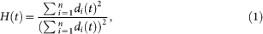where di(t) denotes the outstanding debt of borrower i at time t. When the debt is equally distributed among n institutions, H = 1/n. In contrast, when all the institutions except one have zero debt, then H = 1. Thus, the inverse of H is a measures of the number of leading borrowers. For instance, in the case of 3 institutions with debt values equal to 0.8, 0.1, 0.1, the Herfindhal index is H = 0.82 + 0.12 + 0.12 = 0.66 and H−1 = 1.5.

The higher the debt (relative to the market capitalization of an institution), the weaker it is the institution, financially speaking. Thus, the ratio φi(t) = di/ei captures its financial fragility at time t. The following weighted average gives then a measure of fragility of the entire FED credit portfolio: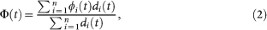The debt-weighted average ensures that the fragility values of the institutions with larger debt count more.

### DebtRank. Methodology

We introduce a directed network in which the nodes represent institutions and the links represent financial dependencies. We denote the amount invested by i in the funding of j as Aij. Thus, A is the weighted adjacency matrix of the investment network. The total value of the asset invested by i in funding activities is Ai = ΣlAil. We denote by Ei the capital of i (more precisely, the so-called “tier 1 capital”), which represents the capital buffer of i against shocks11,12. When Ei ≤ γ the firm defaults, where γ is a positive threshold. Further details on the structure of the balance sheet in relation to a financial network can be found in previous work17. If the node i defaults, the node j faces a loss of Aji (we exclude, in the short run, any recovery of the assets invested in i) and the node j also defaults if Aji > Ej. We will account later on for the case of the node j not defaulting but going only under distress. We define the impact of i on j as Wij = min{1, Aji/Ej}. Thus, if the loss exceeds capital, the impact is 1. Notice that the matrix W is, in general, neither column-stochastic nor row-stochastic. We further take into account the economic value of the impact of i on j by multiplying the impact by the relative economic value of the node j, vj = AjlAl (other proxies could be taken for vj). The value of the impact of i on its neighbours is then Ii = ΣjWijvj. It measures the fraction of economic value in the network that is impacted by i directly.

We now want to take into account the impact of i on its indirect successors, that is, the nodes that can be reached from i and are at distance 2 or more. In analogy to the feedback centrality we could define the impact in terms of the following recursive equation Ii = ΣjWijvj + βΣjWijIj, where the second term accounts for the indirect impact via the neighbours. The parameter β < 1 is a dampening factor. In vector notation, we have I = Wv + βWI, which yields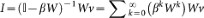, as long as the largest eigenvalue of W is smaller than 1/β.

One result of the graph theory is that in the power k of the adjacency matrix the element ij amounts to the number of walks of length k from i to j. In the case of a weighted matrix, it amounts to a sum across the walks of length k from i to j of the product of the weights along each walk15. Longer walks contribute less to the centrality because of the dampening factor βk. However, if two nodes i and j are connected in a cycle (Wij > 0 and Wji > 0), the impact of node i to j hits back on i and keeps cycling an infinite number of times (although with dampening). A single reverberation of the impact of i back to itself is realistic and mathematically acceptable. Further reverberations lead instead to an inconsistency because the impact could become larger than one. The reason is that if the impact walks its way several times through a cycle, then we are counting the impact of a node on another one more than once. The same problem applies also to any cycle not involving i, but located downstream of i in the network. Removing the cycles altogether from the network and considering its corresponding acyclic graph would remove entirely the reverberation and cut many links, thus strongly underestimating the impact.

In contrast, in this paper, we propose the following approach. We keep the network as it is, but we exclude the walks in which one or more edges are repeated. A convenient way to do this (a sufficient condition) is to introduce the following process. To each node we associate two state variables. hi is a continuous variable with hi [0, 1]. Instead, si is a discrete variable with 3 possible states, undistressed, distressed, inactive: si {U, D, I}. Denoting by Sf the set of nodes in distress at time 1, the initial conditions are: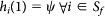;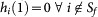and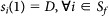;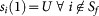. The parameter ψ measures the initial level of distress: ψ [0, 1], with ψ = 1 meaning default. The dynamics is defined as follows,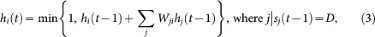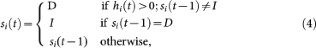for all i and for t> = 2, where all variables hi are first updated in parallel, followed by un update in parallel of all variables si. After a finite number of steps T the dynamics stops and all the nodes in the network are either in state U or I. The intuition is that a nodes goes in distress when a predecessor just went in distress and so recursively. The fraction of propagated distress is given by the impact matrix Wij. Because Wij ≤ 1 the longer the path from the node i initially in distress and node j, the smaller is the indirect impact on j. Notice that a node that goes in the D state, will move to the I state one step later. This means that if there is a cycle of length 2 the node will not be able to propagate impact to its successor more than once. This condition satisfies the requirement, mentioned earlier, of excluding the walks in which an edge is repeated. An illustration on a simple example is provided in the SI, Section 3.2.1.

The DebtRank of the set Sf is then defined as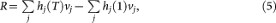i.e., R measures the distressed induced in the system, excluding the initial distress. If Sf is a single node the DebtRank measures the systemic impact of the node on the network. In this case, it is of interest to set ψ = 1 and to see the impact of a defaulting node. If Sf is a set of nodes it can be interesting to compute the impact of a small shock on the group. Indeed, while it is trivial that the default of a large group would cause the default of the whole network, it is not trivial to anticipate the effect of a little distress acting on the whole group.

### DebtRank. Empirical application

The values of the credit exposures are unknown. However, the values of investments of each node j in the equity of i, Zji, are available. We then take them as a starting point to construct a proxy of the impact of node i. We normalise their values,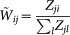. We further rescale them with respect to the maximum by means of an impact scaling factor, denoted as α ≤ 1,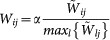. In this way, the maximum impact on j across the nodes l equals α. For instance if α = 0.2, j can withstand the default of 1/0.2 = 5 or more counterparties before defaulting itself: a quite conservative scenario. Notice that the value α = 0.2 is the average ratio Aji/Ei that was observed at the end of 2007 in the Brazilian interbank network, the only case for which this information is published or accessible11. Therefore, we also run the experiments with α = 0.2. One could of course investigate other values of α. Moreover, α could also be made heterogeneous. Finally, we want to account for the fact that the market capitalization of the largest borrowers on the stock market decreased significantly in the first half of the time span of the data. We do so by rescaling the impact of a node on another accordingly, with the constraint that it does not exceed one. The final expression used for the impact is therefore,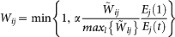.

### Bow-tie

A bow-tie architecture is a directed graph consisting of the following parts. The core is a strongly connected component (SCC). The IN consists of all nodes that can reach the SCC directly or indirectly via one or more directed paths. The OUT consists of all nodes that can be reached from the SCC directly or indirectly via one or more directed path. The Tubes and Tendrils (TT) consist of all nodes that are not in the SCC and are either reachable from the IN or/and can reach the OUT.